Courses

# Solutions of Refraction of Light (Page No- 256) - Physics By Lakhmir Singh, Class 10 Class 10 Notes | EduRev

## Class 10 : Solutions of Refraction of Light (Page No- 256) - Physics By Lakhmir Singh, Class 10 Class 10 Notes | EduRev

The document Solutions of Refraction of Light (Page No- 256) - Physics By Lakhmir Singh, Class 10 Class 10 Notes | EduRev is a part of the Class 10 Course Class 10 Physics Solutions By Lakhmir Singh & Manjit Kaur.
All you need of Class 10 at this link: Class 10

Lakhmir Singh Physics Class 10 Solutions Page No:256

Question 3: An object is placed at a distance of 4 cm from a concave lens of focal length 12 cm. Find the position and nature of the image.

Solution :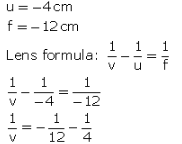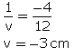Image is formed 3 cm infrcnt of the concave lens.

Image is virtual and erect.

Question 4: A concave lens of focal length 15 cm forms an image 10 cm from the lens. How far is the object placed from the lens ? Draw the ray-diagram.

Solution :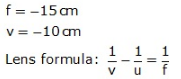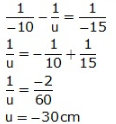Object is at 30 cm from the concave lens (on left side).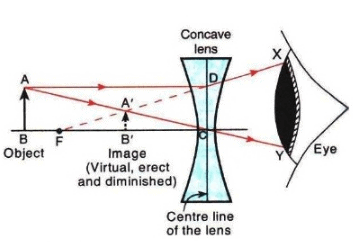Here, OB = 30cm
OF = 15cm
OB' = 10cm

Question 5: An object 60 cm from a lens gives a virtual image at a distance of 20 cm in front of the lens. What is the focal length of the lens ? Is the lens converging or diverging ?

Solution :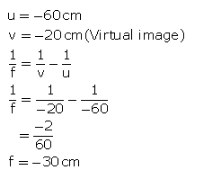The lens is diverging because the focal length is negative.

Question 6: A concave lens of 20 cm focal length forms an image 15 cm from the lens. Compute the object distance.

Solution :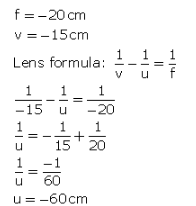Object distance is 60 cm towards the left of the lens.

Question 7: A concave lens has focal length 15 cm. At what distance should the object from the lens be placed so that it forms an image at 10 cm from the lens ? Also find the magnification produced by the lens.

Solution :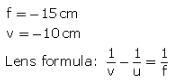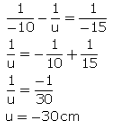Object should be placed at a distance of 60 cm on the left side of the lens.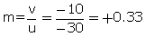Question 8: Calculate the image distance for an object of height 12 mm at a distance of 0.20 m from a concave lens of focal length 0.30 m, and state the nature and size of the image.

Solution :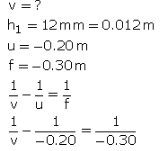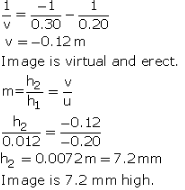Question 9: A concave lens has a focal length of 20 cm. At what distance from the lens a 5 cm tall object be placed so that it forms an image at 15 cm from the lens ? Also calculate the size of the image formed.

Solution :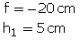v =-15cm (Concave lens forms virtual image)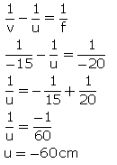Object should be placed 60 cm to the left of the lens.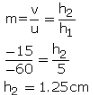Image formed is 1.25 cm high.

Question 10: An object is placed 20 cm from (a) a converging lens, and (b) a diverging lens, of focal length 15 cm. Calculate the image position and magnification in each case.

Solution :

u = - 20 cm
(a) f = 15cm (for converging lens)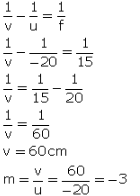(b) f = -15cm (for diverging lens)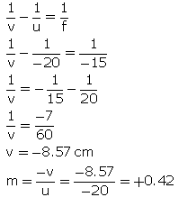Question 11: A 2.0 cm tall object is placed 40 cm from a diverging lens of focal length 15 cm. Find the position and size of the image.

Solution : h1 = 2 cm
u = - 40 cm
f = -15cm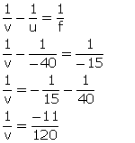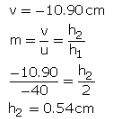Question 12: (a) Find the position and size of the virtual image formed when an object 2 cm tall is placed 20 cm from :
(i) a diverging lens of focal length 40 cm.
(ii) a converging lens of focal length 40 cm.
(b) Draw labelled ray diagrams to show the formation of images in cases (i) and (ii)above (The diagrams may not be according to scale).

Solution : h1 = 2 cm
u = - 20 cm
f = -40cm (Diverging lens)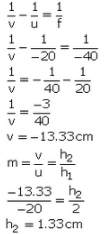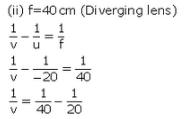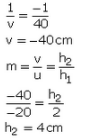(b) Formation of image in case (I):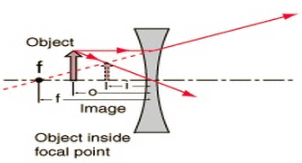Formation of image in case (ii):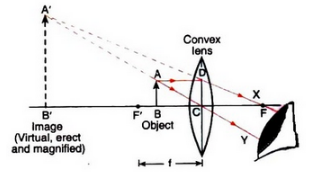Question 13: (a) A small object is placed 150 mm away from a diverging lens of focal length 100 mm.
(i) Copy the figure below and draw rays to show how an image is formed by the lens.
(ii) Calculate the distance of the image from the lens by using the lens formula.
(b) The diverging lens in part (a) is replaced by a converging lens also of focal length 100 mm. The object remains in the same position and an image is formed by the converging lens. Compare two properties of this image with those of the image formed by the diverging lens in part (a).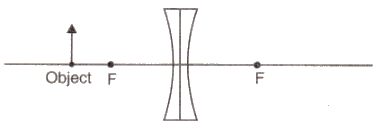Solution :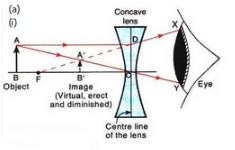Here, OB = 150 mm
OF = 100 mm

(ii) u = -150 mmm
f = -100 mm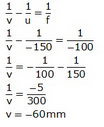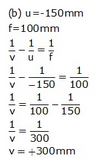The image formed by converging lens is real, inverted and magnified (2 times). It is formed behind the converging lens. On the other hand, the image formed by diverging lens is virtual, erect and diminished. It is formed in front of the diverging lens.

Offer running on EduRev: Apply code STAYHOME200 to get INR 200 off on our premium plan EduRev Infinity!

94 docs

,

,

,

,

,

,

,

,

,

,

,

,

,

,

,

,

,

,

,

,

,

,

,

,

;